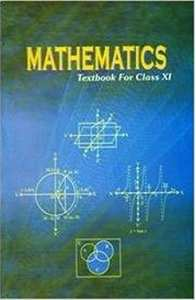CBSE (Commerce) Class 11CBSE
Share

NCERT solutions for Class 11 Mathematics chapter 4 - Principle of Mathematical Induction

Mathematics Textbook for Class 11

NCERT Mathematics Class 11Chapter 4: Principle of Mathematical Induction

Chapter 4: Principle of Mathematical Induction solutions [Pages 94 - 95]

Q 1 | Page 94

Prove the following by using the principle of mathematical induction for all n ∈ N

1 + 3 + 3^2 + ... + 3^(n – 1) =((3^n -1))/2

Q 2 | Page 94

Prove the following by using the principle of mathematical induction for all n ∈ N

1^3 +  2^3 + 3^3 + ... + n^3 = ((n(n+1))/2)^2

Q 3 | Page 94

Prove the following by using the principle of mathematical induction for all n ∈ N

1+ 1/((1+2)) + 1/((1+2+3)) +...+ 1/((1+2+3+...n)) = (2n)/(n +1)
Q 4 | Page 94

Prove the following by using the principle of mathematical induction for all n ∈ N: 1.2.3 + 2.3.4 + … + n(n + 1) (n + 2)  = (n(n+1)(n+2)(n+3))/(4(n+3))

Q 5 | Page 94

Prove the following by using the principle of mathematical induction for all n ∈ N

1.3 + 2.3^3 + 3.3^3  +...+ n.3^n = ((2n -1)3^(n+1) + 3)/4
Q 6 | Page 94

Prove the following by using the principle of mathematical induction for all n ∈ N

1.2 + 2.3 + 3.4+ ... + n(n+1) = [(n(n+1)(n+2))/3]

Q 7 | Page 94

Prove the following by using the principle of mathematical induction for all n ∈ N

1.3 + 3.5 + 5.7 + ...+(2n -1)(2n + 1) = (n(4n^2 + 6n -1))/3
Q 8 | Page 94

Prove the following by using the principle of mathematical induction for all n ∈ N: 1.2 + 2.22 + 3.22 + … + n.2n = (n – 1) 2n+1 + 2

Q 9 | Page 94

Prove the following by using the principle of mathematical induction for all n ∈ N: 1/2 + 1/4 + 1/8 + ... + 1/2^n = 1 - 1/2^n

Q 10 | Page 94

Prove the following by using the principle of mathematical induction for all n ∈ N

1/2.5 + 1/5.8 + 1/8.11 + ... + 1/((3n - 1)(3n + 2)) = n/(6n + 4)
Q 11 | Page 94

Prove the following by using the principle of mathematical induction for all n ∈ N

1/1.2.3 + 1/2.3.4 + 1/3.4.5 + ...+ 1/(n(n+1)(n+2)) = (n(n+3))/(4(n+1) (n+2))
Q 12 | Page 95

Prove the following by using the principle of mathematical induction for all n ∈ N

a + ar + ar^2 + ... + ar^(n -1) = (a(r^n - 1))/(r -1)
Q 13 | Page 95

Prove the following by using the principle of mathematical induction for all n ∈ N

(1+3/1)(1+ 5/4)(1+7/9)...(1 + ((2n + 1))/n^2) = (n + 1)^2

Q 14 | Page 95

Prove the following by using the principle of mathematical induction for all n ∈ N

(1+ 1/1)(1+ 1/2)(1+ 1/3)...(1+ 1/n) = (n + 1)

Q 15 | Page 95

Prove the following by using the principle of mathematical induction for all n ∈ N

1^2 + 3^2 + 5^2 + ... + (2n -1)^2 = (n(2n - 1) (2n + 1))/3
Q 16 | Page 95

Prove the following by using the principle of mathematical induction for all n ∈ N

1/1.4 + 1/4.7 + 1/7.10 + ... + 1/((3n - 2)(3n + 1)) = n/((3n + 1))

Q 17 | Page 95

Prove the following by using the principle of mathematical induction for all n ∈ N

1/3.5 + 1/5.7 + 1/7.9 + ...+ 1/((2n + 1)(2n +3)) = n/(3(2n +3))
Q 18 | Page 95

Prove the following by using the principle of mathematical induction for all n ∈ N: 1+2+ 3+...+n<1/8(2n +1)^2

Q 19 | Page 95

Prove the following by using the principle of mathematical induction for all n ∈ Nn (n + 1) (n + 5) is a multiple of 3.

Q 20 | Page 95

Prove the following by using the principle of mathematical induction for all n ∈ N: 102n – 1 + 1 is divisible by 11

Q 21 | Page 95

Prove the following by using the principle of mathematical induction for all n ∈ Nx2n – y2n is divisible by x y.

Q 22 | Page 95

Prove the following by using the principle of mathematical induction for all n ∈ N: 32n + 2 – 8n– 9 is divisible by 8.

Q 23 | Page 95

Prove the following by using the principle of mathematical induction for all n ∈ N: 41n – 14n is a multiple of 27.

Q 24 | Page 95

Prove the following by using the principle of mathematical induction for all n ∈ N (2+7) < (n + 3)2

Chapter 4: Principle of Mathematical Induction

NCERT Mathematics Class 11NCERT solutions for Class 11 Mathematics chapter 4 - Principle of Mathematical Induction

NCERT solutions for Class 11 Maths chapter 4 (Principle of Mathematical Induction) include all questions with solution and detail explanation. This will clear students doubts about any question and improve application skills while preparing for board exams. The detailed, step-by-step solutions will help you understand the concepts better and clear your confusions, if any. Shaalaa.com has the CBSE Mathematics Textbook for Class 11 solutions in a manner that help students grasp basic concepts better and faster.

Further, we at Shaalaa.com are providing such solutions so that students can prepare for written exams. NCERT textbook solutions can be a core help for self-study and acts as a perfect self-help guidance for students.

Concepts covered in Class 11 Mathematics chapter 4 Principle of Mathematical Induction are Motivation, Principle of Mathematical Induction.

Using NCERT Class 11 solutions Principle of Mathematical Induction exercise by students are an easy way to prepare for the exams, as they involve solutions arranged chapter-wise also page wise. The questions involved in NCERT Solutions are important questions that can be asked in the final exam. Maximum students of CBSE Class 11 prefer NCERT Textbook Solutions to score more in exam.

Get the free view of chapter 4 Principle of Mathematical Induction Class 11 extra questions for Maths and can use Shaalaa.com to keep it handy for your exam preparation

S